# RD Sharma Solutions for Class 10 Maths Chapter 15 Areas Related To Circles Exercise 15.1

The problem of finding perimeters and areas related to circular figures is of great practical importance. This exercise contains problems on finding the perimeter and area of a circle as well as the area enclosed by two concentric circles. To access detailed solutions to problems, the RD Sharma Solutions Class 10 is available for students for the purpose of clearing doubts. Downloadable format of the RD Sharma Solutions for Class 10 Maths Chapter 15 Areas Related To Circles Exercise 15.1 PDF is provided below.

## RD Sharma Solutions for Class 10 Maths Chapter 15 Areas Related To Circles Exercise 15.1 Download PDF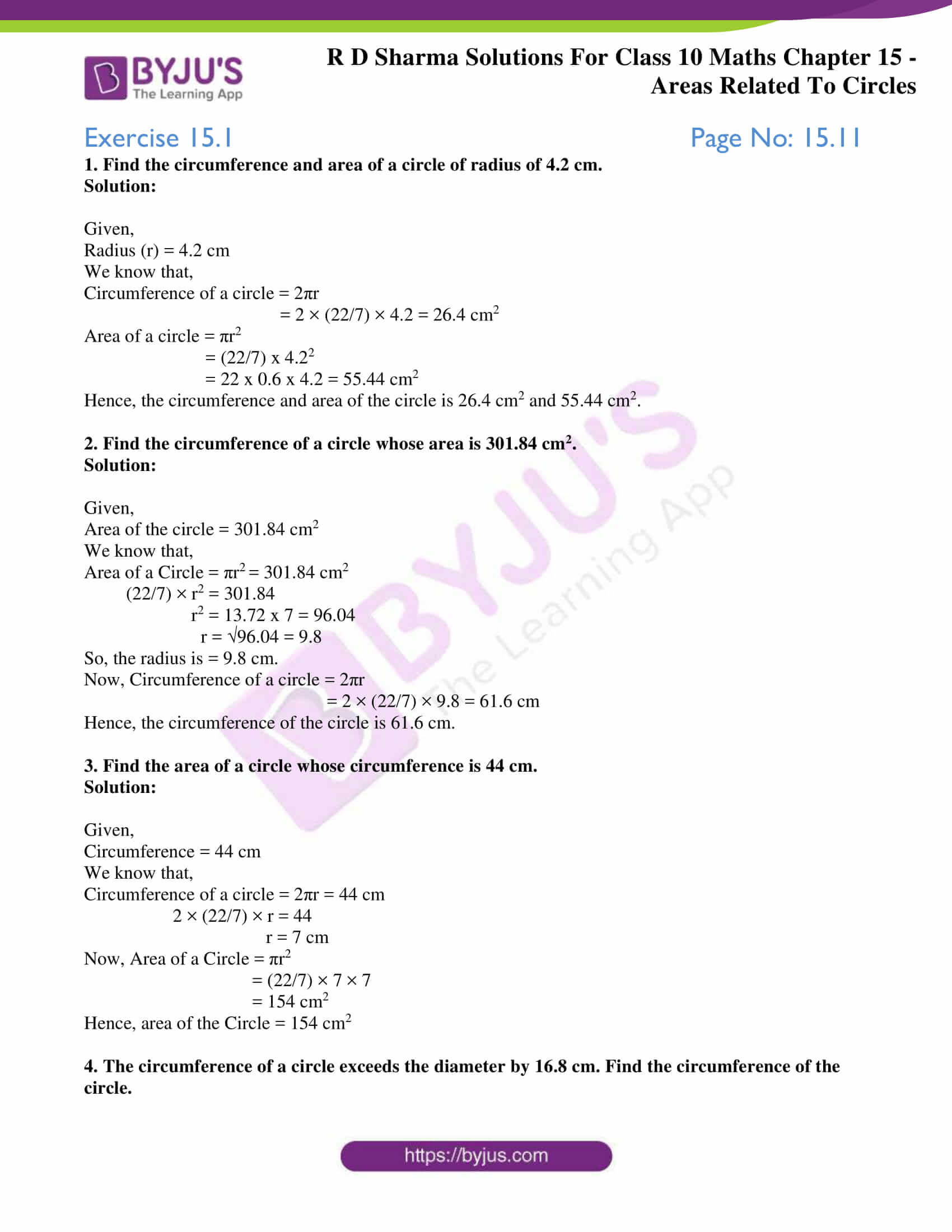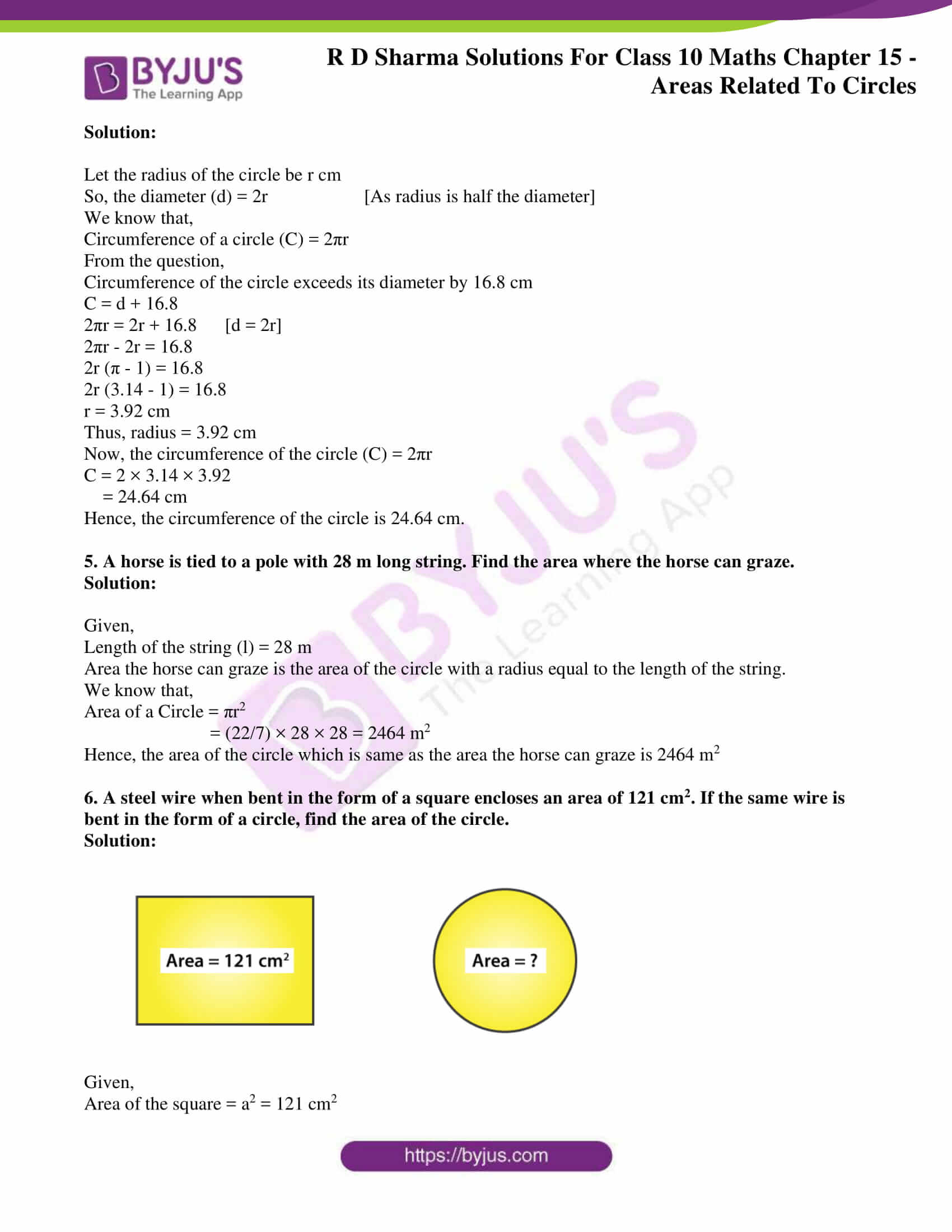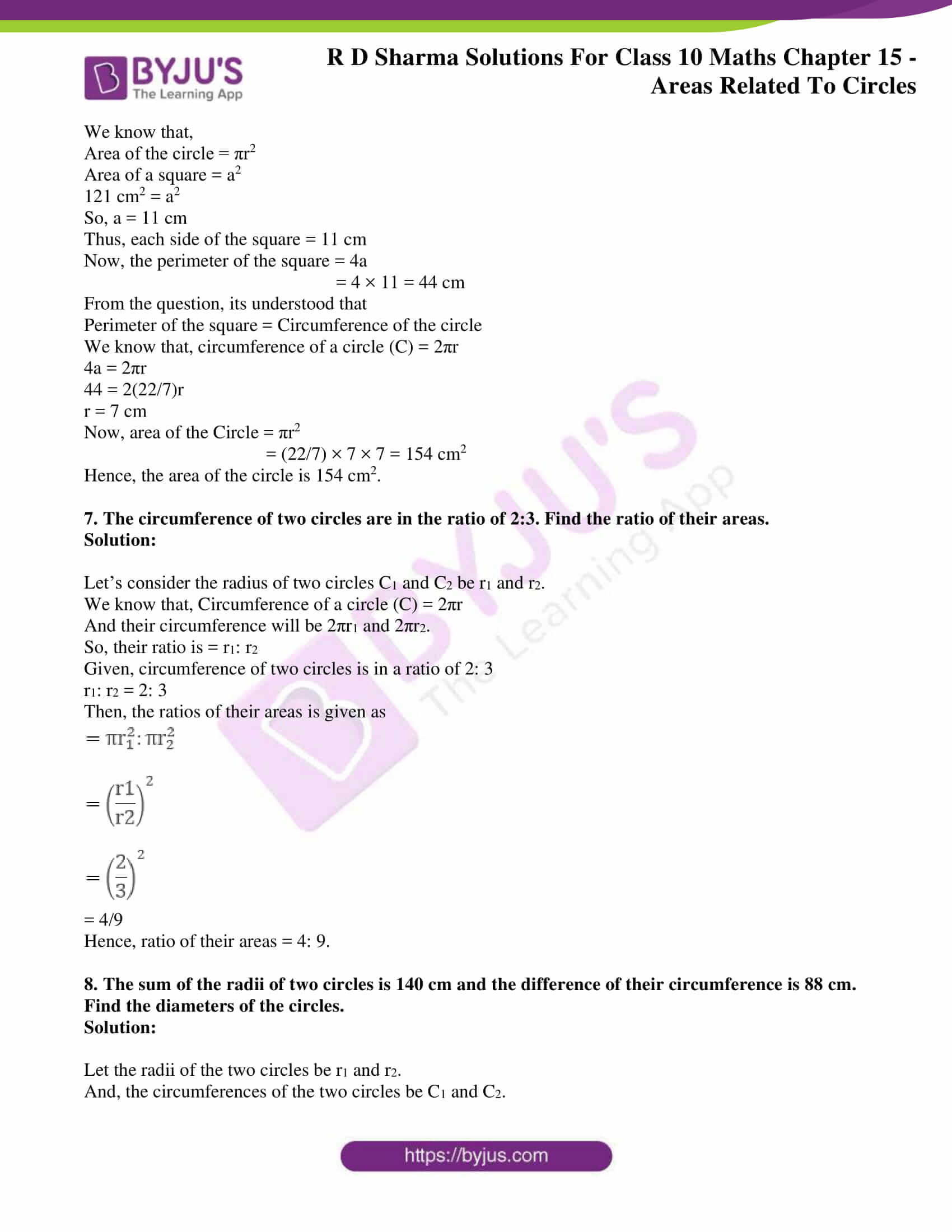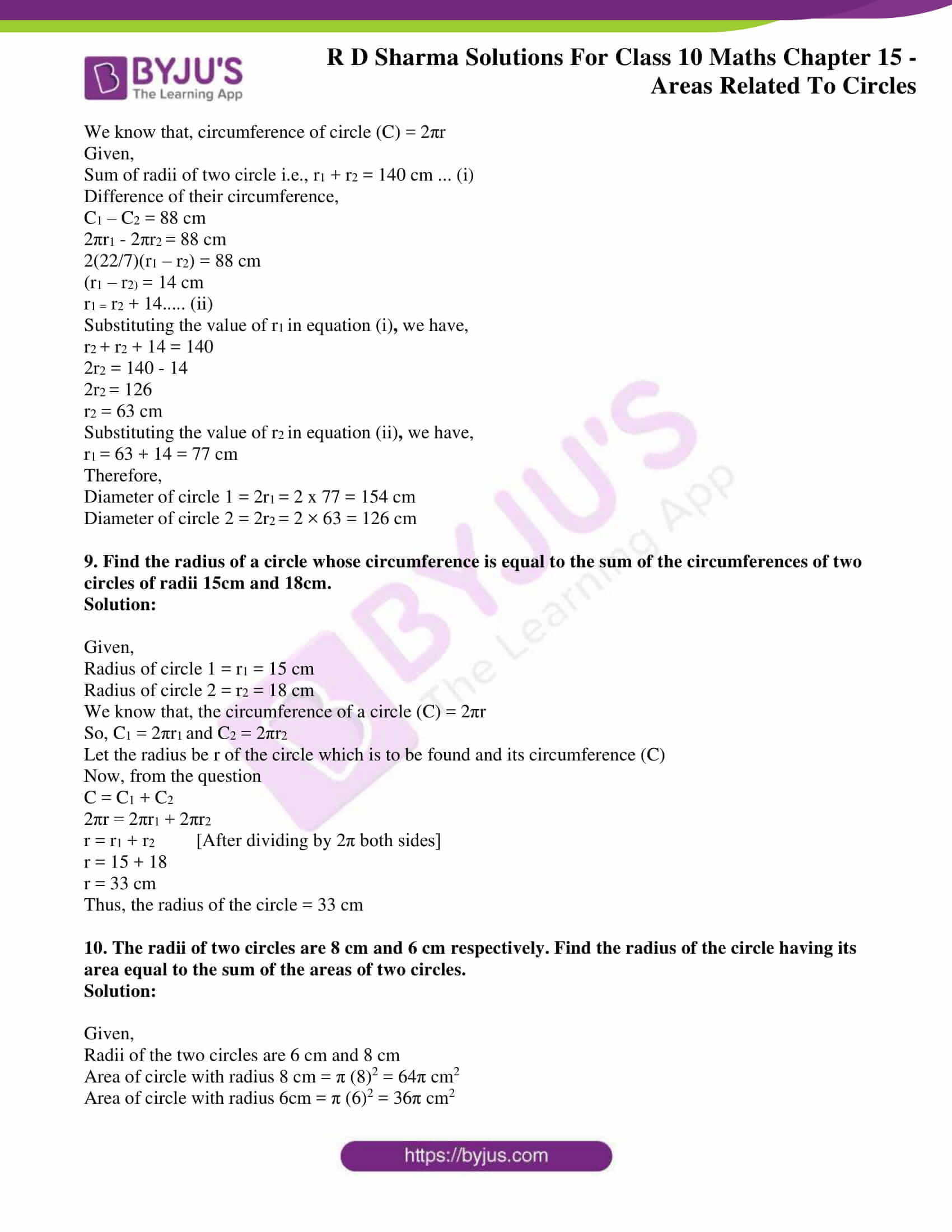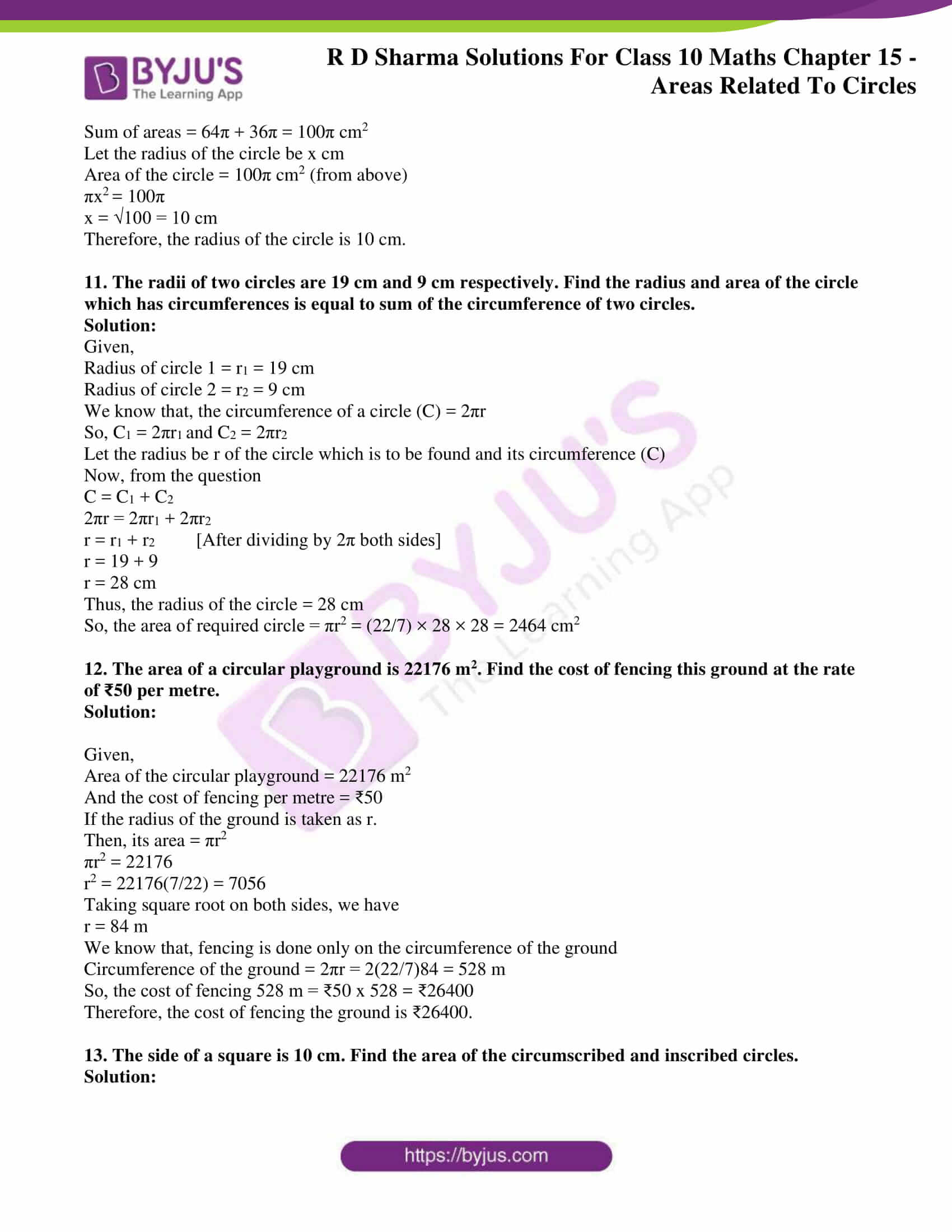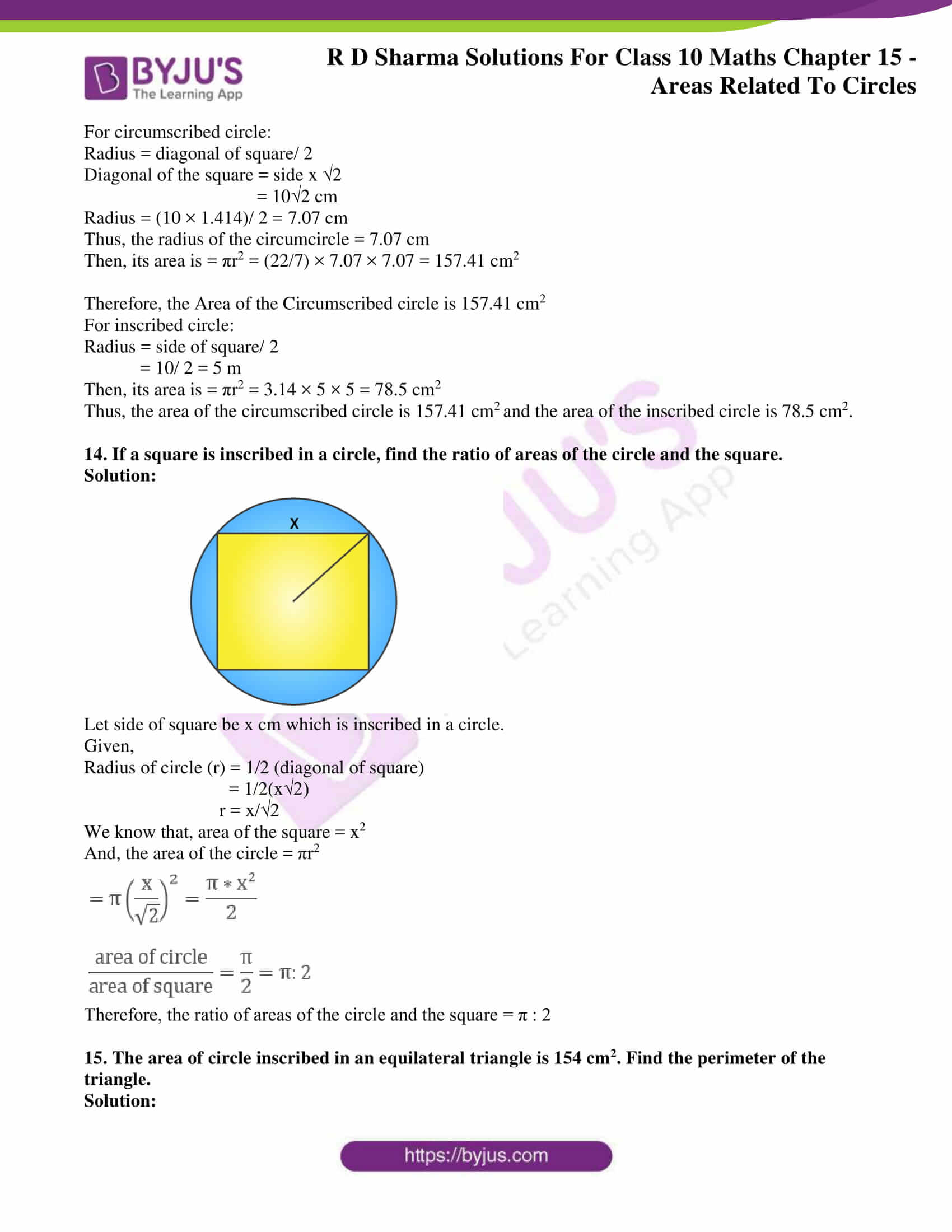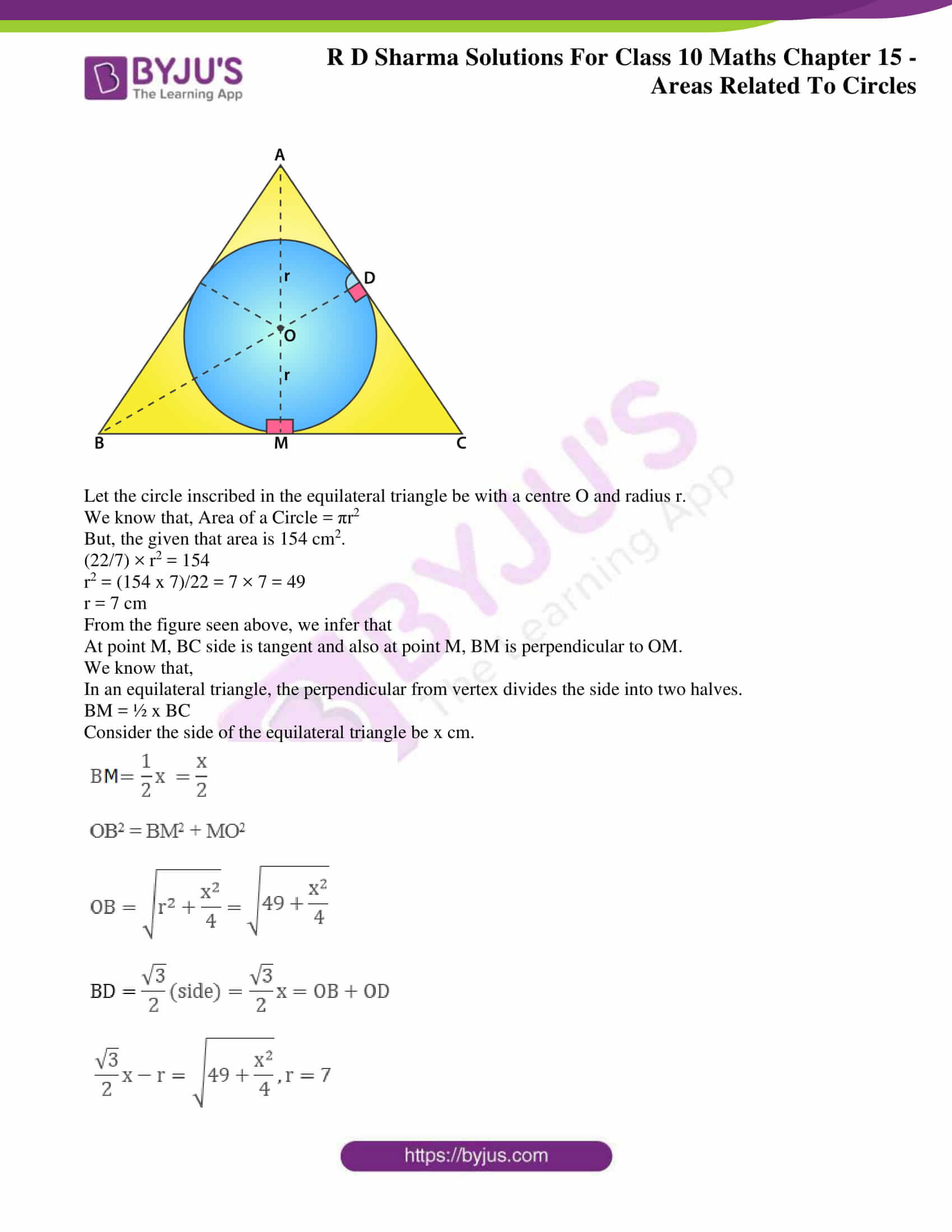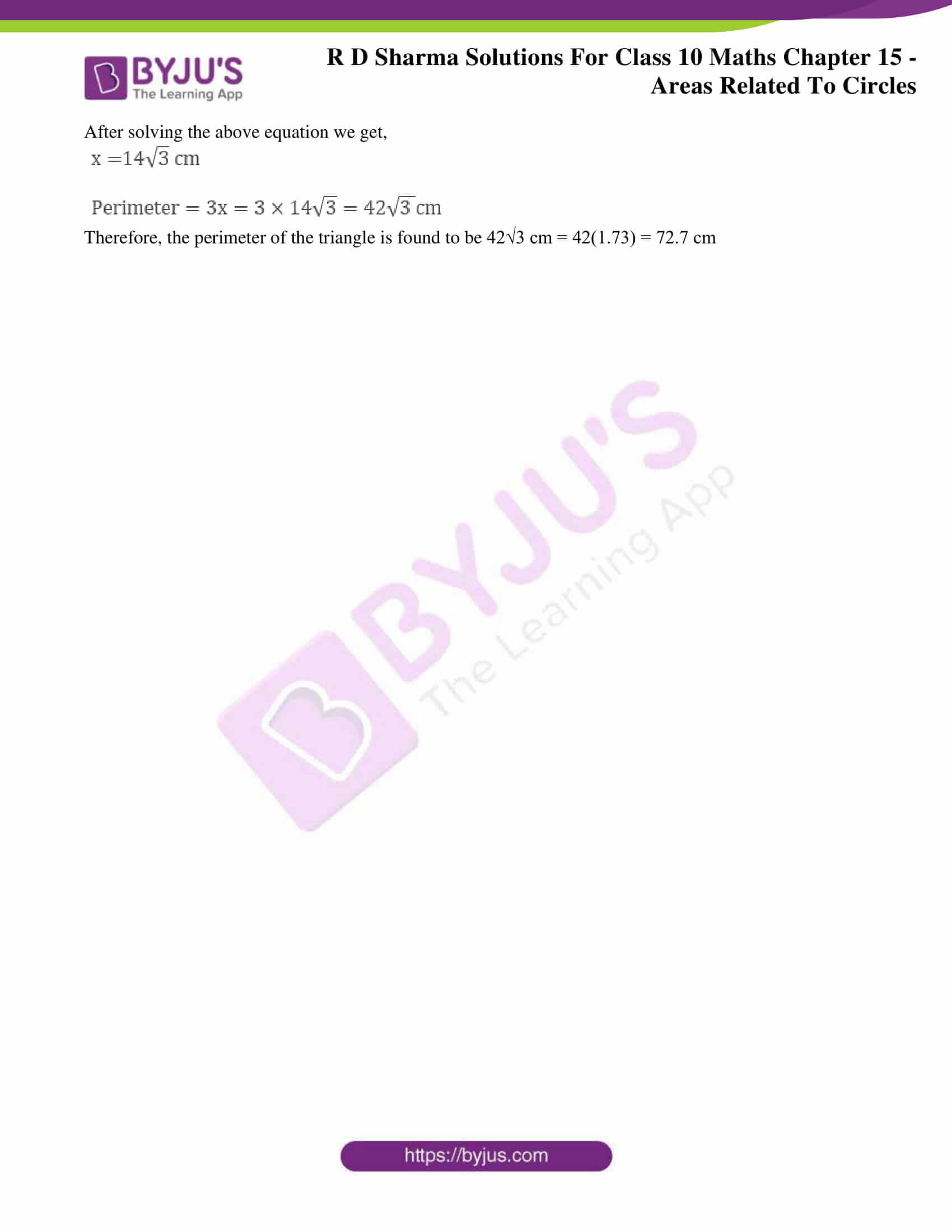### Access RD Sharma Solutions for Class 10 Maths Chapter 15 Areas Related To Circles Exercise 15.1

1. Find the circumference and area of a circle of radius of 4.2 cm.

Solution:

Given,

We know that,

Circumference of a circle = 2πr

= 2 × (22/7) × 4.2 = 26.4 cm2

Area of a circle = πr2

= (22/7) x 4.22

= 22 x 0.6 x 4.2 = 55.44 cm2

Hence, the circumference and area of the circle is 26.4 cm2 and 55.44 cm2.

2. Find the circumference of a circle whose area is 301.84 cm2

Solution:

Given,

Area of the circle = 301.84 cm2

We know that,

Area of a Circle = πr2 = 301.84 cm2

(22/7) × r2 = 301.84

r2 = 13.72 x 7 = 96.04

r = √96.04 = 9.8

So, the radius is = 9.8 cm.

Now, Circumference of a circle = 2πr

= 2 × (22/7) × 9.8 = 61.6 cm

Hence, the circumference of the circle is 61.6 cm.

3. Find the area of a circle whose circumference is 44 cm.

Solution:

Given,

Circumference = 44 cm

We know that,

Circumference of a circle = 2πr = 44 cm

2 × (22/7) × r = 44

r = 7 cm

Now, Area of a Circle = πr2

= (22/7) × 7 × 7

= 154 cm2

Hence, area of the Circle = 154 cm2

4. The circumference of a circle exceeds the diameter by 16.8 cm. Find the circumference of the circle.

Solution:

Let the radius of the circle be r cm

So, the diameter (d) = 2r [As radius is half the diameter]

We know that,

Circumference of a circle (C) = 2πr

From the question,

Circumference of the circle exceeds its diameter by 16.8 cm

C = d + 16.8

2πr = 2r + 16.8      [d = 2r]

2πr – 2r = 16.8

2r (π – 1) = 16.8

2r (3.14 – 1) = 16.8

r = 3.92 cm

Now, the circumference of the circle (C) = 2πr

C = 2 × 3.14 × 3.92

= 24.64 cm

Hence, the circumference of the circle is 24.64 cm.

5. A horse is tied to a pole with 28 m long string. Find the area where the horse can graze.

Solution:

Given,

Length of the string (l) = 28 m

Area the horse can graze is the area of the circle with a radius equal to the length of the string.

We know that,

Area of a Circle = πr2

= (22/7) × 28 × 28 = 2464 m2

Hence, the area of the circle which is same as the area the horse can graze is 2464 m2

6. A steel wire when bent in the form of a square encloses an area of 121 cm2. If the same wire is bent in the form of a circle, find the area of the circle.

Solution: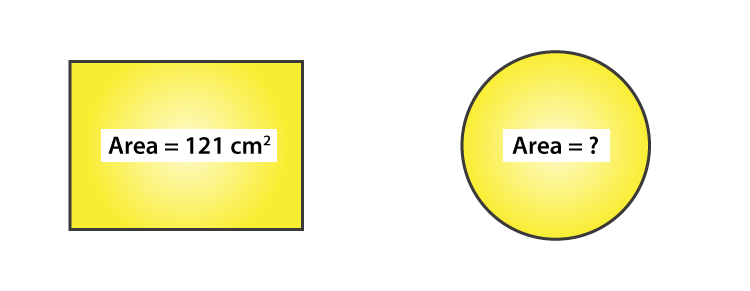Given,

Area of the square = a2 = 121 cm2

We know that,

Area of the circle = πr2

Area of a square = a2

121 cm2 = a2

So, a = 11 cm

Thus, each side of the square = 11 cm

Now, the perimeter of the square = 4a

= 4 × 11 = 44 cm

From the question, its understood that

Perimeter of the square = Circumference of the circle

We know that, circumference of a circle (C) = 2πr

4a = 2πr

44 = 2(22/7)r

r = 7 cm

Now, area of the Circle = πr2

= (22/7) × 7 × 7 = 154 cm2

Hence, the area of the circle is 154 cm2.

7. The circumference of two circles are in the ratio of 2:3. Find the ratio of their areas.

Solution:

Let’s consider the radius of two circles C1 and C2 be r1 and r2.

We know that, Circumference of a circle (C) = 2πr

And their circumference will be 2πr1 and 2πr2.

So, their ratio is = r1: r2

Given, circumference of two circles is in a ratio of 2: 3

r1: r2 = 2: 3

Then, the ratios of their areas is given as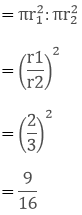= 4/9

Hence, ratio of their areas = 4: 9.

8. The sum of the radii of two circles is 140 cm and the difference of their circumference is 88 cm. Find the diameters of the circles.

Solution:

Let the radii of the two circles be r1 and r2.

And, the circumferences of the two circles be C1 and C2.

We know that, circumference of circle (C) = 2πr

Given,

Sum of radii of two circle i.e., r1 + r2 = 140 cm … (i)

Difference of their circumference,

C1 – C2 = 88 cm

2πr1 – 2πr= 88 cm

2(22/7)(r1 – r2) = 88 cm

(r1 – r2) = 14 cm

r1 = r2 + 14….. (ii)

Substituting the value of rin equation (i)we have,

r+ r2 + 14 = 140

2r2 = 140 – 14

2r= 126

r2 = 63 cm

Substituting the value of rin equation (ii)we have,

r= 63 + 14 = 77 cm

Therefore,

Diameter of circle 1 = 2r= 2 x 77 = 154 cm

Diameter of circle 2 = 2r= 2 × 63 = 126 cm

9. Find the radius of a circle whose circumference is equal to the sum of the circumferences of two circles of radii 15cm and 18cm.

Solution:

Given,

Radius of circle 1 = r1 = 15 cm

Radius of circle 2 = r2 = 18 cm

We know that, the circumference of a circle (C) = 2πr

So, C1 = 2πr1 and C2 = 2πr2

Let the radius be r of the circle which is to be found and its circumference (C)

Now, from the question

C = C1 + C2

2πr = 2πr1 + 2πr2

r = r1 + r2 [After dividing by 2π both sides]

r = 15 + 18

r = 33 cm

Thus, the radius of the circle = 33 cm

10. The radii of two circles are 8 cm and 6 cm respectively. Find the radius of the circle having its area equal to the sum of the areas of two circles.

Solution:

Given,

Radii of the two circles are 6 cm and 8 cm

Area of circle with radius 8 cm = π (8)2 = 64π cm2

Area of circle with radius 6cm = π (6)2 = 36π cm2

Sum of areas = 64π + 36π = 100π cm2

Let the radius of the circle be x cm

Area of the circle = 100π cm2 (from above)

πx= 100π

x = √100 = 10 cm

Therefore, the radius of the circle is 10 cm.

11. The radii of two circles are 19 cm and 9 cm respectively. Find the radius and area of the circle which has circumferences is equal to sum of the circumference of two circles.

Solution:

Given,

Radius of circle 1 = r1 = 19 cm

Radius of circle 2 = r2 = 9 cm

We know that, the circumference of a circle (C) = 2πr

So, C1 = 2πr1 and C2 = 2πr2

Let the radius be r of the circle which is to be found and its circumference (C)

Now, from the question

C = C1 + C2

2πr = 2πr1 + 2πr2

r = r1 + r2 [After dividing by 2π both sides]

r = 19 + 9

r = 28 cm

Thus, the radius of the circle = 28 cm

So, the area of required circle = πr2 = (22/7) × 28 × 28 = 2464 cm2

12. The area of a circular playground is 22176 m2. Find the cost of fencing this ground at the rate of ₹50 per metre.

Solution:

Given,

Area of the circular playground = 22176 m2

And the cost of fencing per metre = ₹50

If the radius of the ground is taken as r.

Then, its area = πr2

πr2 = 22176

r2 = 22176(7/22) = 7056

Taking square root on both sides, we have

r = 84 m

We know that, fencing is done only on the circumference of the ground

Circumference of the ground = 2πr = 2(22/7)84 = 528 m

So, the cost of fencing 528 m = ₹50 x 528 = ₹26400

Therefore, the cost of fencing the ground is ₹26400.

13. The side of a square is 10 cm. Find the area of the circumscribed and inscribed circles.

Solution:

For circumscribed circle:

Radius = diagonal of square/ 2

Diagonal of the square = side x √2

= 10√2 cm

Radius = (10 × 1.414)/ 2 = 7.07 cm

Thus, the radius of the circumcircle = 7.07 cm

Then, its area is = πr2 = (22/7) × 7.07 × 7.07 = 157.41 cm

Therefore, the Area of the Circumscribed circle is 157.41 cm

For inscribed circle:

Radius = side of square/ 2

= 10/ 2 = 5 m

Then, its area is = πr2 = 3.14 × 5 × 5 = 78.5 cm

Thus, the area of the circumscribed circle is 157.41 cmand the area of the inscribed circle is 78.5 cm2.

14. If a square is inscribed in a circle, find the ratio of areas of the circle and the square.

Solution: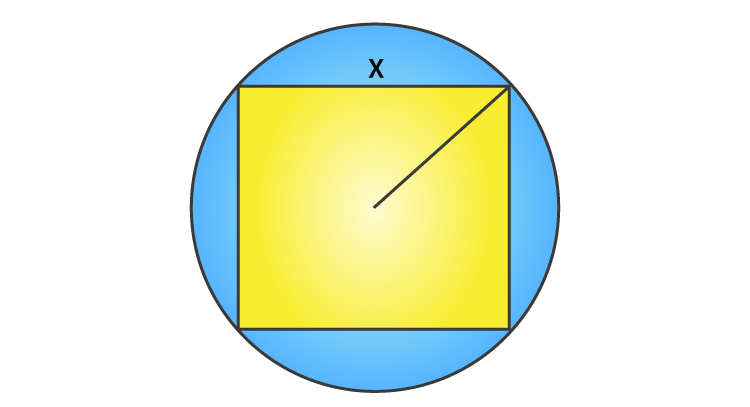Let side of square be x cm which is inscribed in a circle.

Given,

Radius of circle (r) = 1/2 (diagonal of square)

= 1/2(x√2)

r = x/√2

We know that, area of the square = x2

And, the area of the circle = πr2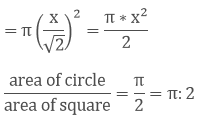Therefore, the ratio of areas of the circle and the square = π : 2

15. The area of circle inscribed in an equilateral triangle is 154 cm2. Find the perimeter of the triangle.

Solution: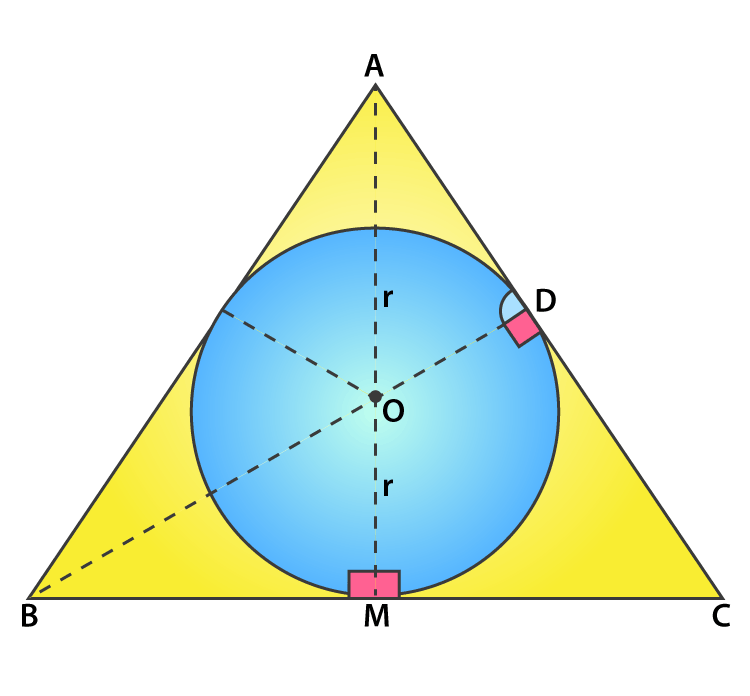Let the circle inscribed in the equilateral triangle be with a centre O and radius r.

We know that, Area of a Circle = πr2

But, the given that area is 154 cm2.

(22/7) × r2 = 154

r2 = (154 x 7)/22 = 7 × 7 = 49

r = 7 cm

From the figure seen above, we infer that

At point M, BC side is tangent and also at point M, BM is perpendicular to OM.

We know that,

In an equilateral triangle, the perpendicular from vertex divides the side into two halves.

BM = ½ x BC

Consider the side of the equilateral triangle be x cm.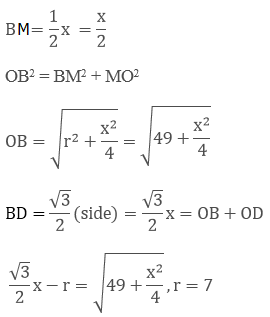After solving the above equation we get,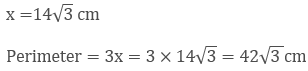Therefore, the perimeter of the triangle is found to be 42√3 cm = 42(1.73) = 72.7 cm